INVERSE TRIGONOMETRIC FUNCTIONS

Example 1:

Using a calculator, find sin -1(0.86) in degrees and radians rounded to two decimal places.

You access sin -1 by first pressing the "2nd" or "Shift" key on your calculator and then pressing the "sin" key.

This calculation will result in approximately 59.32o or 1.04 radians.

Example 2:

Using a calculator, find cos -1(0.87) in degrees and radians rounded to two decimal places.

You access cos -1 by first pressing the "2nd" or "Shift" key on your calculator and then pressing the "cos" key.

This calculation results in approximately 29.54o or 0.52 radians.

Example 3:

Using a calculator, find tan -1(0.59) in degrees and radians rounded to two decimal places.

You access tan -1 by first pressing the "2nd" or "Shift" key on your calculator and then pressing the "tan" key.

This calculation results in approximately 30.54o or 0.53 radians

Example 4:

Compare some trigonometric functions with their inverses.  KEEP YOUR CALCULATOR IN DEGREE MODE!

 Given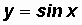letThenGivenletThenPlease note that arcsin(0.5) gives us back an angle of 30o.

Example 5:

Compare some trigonometric functions with their inverses.  KEEP YOUR CALCULATOR IN DEGREE MODE!

 Givenlet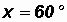Then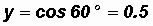GivenletThen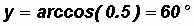Please note that arccos(0.5) gives us back an angle of 60o.

Example 6:

Compare some trigonometric functions with their inverses.  KEEP YOUR CALCULATOR IN DEGREE MODE!

 Givenlet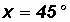ThenGivenletThenPlease note that arctan(1) gives us back an angle of 45o.

Example 7:

Let's compare some more trigonometric functions with their inverses.  KEEP YOUR CALCULATOR IN DEGREE MODE!

 Givenlet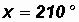ThenGiven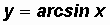letThen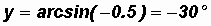Please note that arcsin(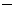0.5) DOES NOT gives us back an angle of 210o.

WHY?

Due to the range restrictions for the inverse sine function to90o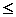y90o, the calculator gives us the angle from the restricted range. That's why the arcsin(0.5) is30o and not 210o.

Example 8:

Compare some trigonometric functions with their inverses.  KEEP YOUR CALCULATOR IN DEGREE MODE!

 GivenletThenGivenlet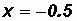Then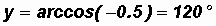Please note that arccos(0.5) DOES NOT gives us back an angle of 240o.

WHY?

Due to the range restrictions for the inverse cosine function to 0oy180o, the calculator gives us the angle from the restricted range. That's why the arccos(0.5) is 120o and not 240o.

Example 9:

Compare some trigonometric functions with their inverses.  KEEP YOUR CALCULATOR IN DEGREE MODE!

 GivenletThen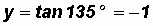GivenletThenPlease note that arctan(1) DOES NOT gives us back an angle of 135o.

WHY?

Due to the range restrictions for the inverse tangent function to90o < y < 90o, the calculator gives us the angle from the restricted range. That's why the arctan(1) is45o and not 135o.